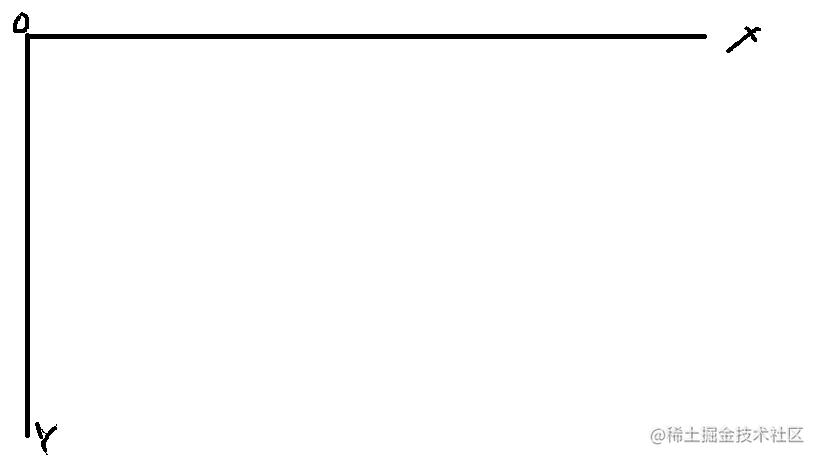# 游离在平面中的像素

上一篇文章中，我们讲解了最基础的粒子动画，实现了在浏览器中跑来跑去的粒子以及讲解了粒子的一些常见特效的原理。

我们把所有的运动公式都封装在了dot类中，虽说实现了我们的当时需求，但是如果引申到一些方向性的东西的时候，就会显得苍白而无力。

## 平面中的指向标（vector2）

向量确实是一个神奇的东西，当坐标系确定了之后，向量不仅仅可以看作是一个坐标点，还可以看做是一段距离，同时还具有方向性，这就对于我们在平面中的定向操作就提供了很大的帮助。

1. 定义一个二维向量类

``````function  vector2(x,y) {
//当前的坐标的x,y
this.x=x;
this.y=y;
}复制代码``````
2. 向量的移动

当`x2``y2`都为0的时候，也就是存在于坐标原点时，相减之后还会是`(x1,y1)`，这也是为什么我说它不仅可以代表一个坐标，还可以代表一段距离

``````vector2.prototype.move = function(vec2) {
this.x+=vec2.x;
this.y+=vec2.y;
};复制代码``````
3. 把向量单位化

单位向量，可以理解成在一段向量上，长度为1的向量

``````vector2.prototype.normalize = function(vec2) {
var x=vec2.x-this.x;
var y=vec2.y-this.y;
//以上是为了将当前坐标转换为原点，从而生成一段新的向量
return {
//返回一个单位向量
x:x/Math.sqrt(x*x+y*y),
y:y/Math.sqrt(x*x+y*y)
}
};复制代码``````

如果对于向量不熟悉的可以去看一看向量的公式，也许你看着会有一点晕，那么我来举一个例子。

`(1，1)`这是一个二维的向量，我可以用它来代表一个坐标，也可以代表它到原点的距离，同时也可以代表从原点到该点的方向。

## 拥有向量的粒子类

1. 当粒子遇上向量

``````function dot(x,y,ax=0,ay=0,color="black") {
//用向量来替代之前的纯坐标
this.site=new vector2(x,y);
//初始时的目标坐标向量既是当前坐标向量
this.end=new vector2(x,y);
//速度全部交由系统来调整
this.vx=0;
this.vy=0；
//加速度受外界力影响
this.ax=ax;
this.ay=ay;
this.color=color;
//设置水平与竖直方向的值，具体作用下面有讲
this.direction={
hor:0,
ver:0
}
this.ctx={};
}复制代码``````

在实现的时候，我们需要知道什么是受我们直接影响的，什么是受我们间接影响的，这样可以可以在实现的实现的时候更具有联动性，把更多的计算交由计算机去处理，可以大大避免一些不必要的误操作。

2. 最终点的设置

``````dot.prototype.setEnd = function(vec2) {
this.end=vec2;
this.vx=this.site.normalize(this.end).x;
this.vy=this.site.normalize(this.end).y;
if(vec2.x>this.site.x){this.direction.hor=1}
if(vec2.x<this.site.x){this.direction.hor=-1}
if(vec2.y>this.site.y){this.direction.ver=-1}
if(vec2.y<this.site.y){this.direction.ver=1}
};复制代码``````

这里会发现我们的方向速度量是取的位移向量的单位向量，这里的原因是`X=VT`，当时间一定时，速度可以视作位移上的单位距离，然后将其方向化，也就获得了方向速度。

这里我将粒子的初始位置与目标位置做了对比，获得了相对而言的上下或者左右，并且使用`1`或者`-1`来分别代替下右跟上左，有部分人不知道这里为什么是下右跟上左，这是因为在屏幕上的y轴的计算是以下方问正方向的，这也为什么我们在绘制的时候是用`(100，100)`而不是`(100,-100)`3. 粒子的移动

``````dot.prototype.move = function() {
this.vy+=this.ay*t;
this.vx+=this.ax*t;
var hor="";
var ver="";
//对取整的方式进行判断
switch (this.direction.hor) {
case 1:
hor='floor';
break;
case 0:
hor='floor';
break;
case -1:
hor='ceil';
default:

break;
}
switch (this.direction.ver) {
case 1:
ver='ceil';
break;
case 0:
ver='floor';
break;
case -1:
ver='floor';
default:

break;
}
if(Math[hor](this.site.x)!=this.end.x||Math[ver](this.site.y)!=this.end.y){
this.site.move(new vector2(this.vx,this.vy));
}
};复制代码``````

移动的基本公式上一节也有基础的介绍，这里直接用的是带加速度的，`v=v0+at`(v0是初速度，v是匀变速t时间之后的速度)

在位置判断的时候我使用了向下取整的方法，是因为在做单位向量的时候会出现带根号的数，为了便于判断，我们就需要对位置的坐标进行取整，这个时候，我们就需要根据粒子点在目标点的方位来决定是向上还是向下取整。

取整之后就可以进行判断是否达到目标位置，如果没有就会继续调用`move`的命令来不断更新坐标位置。

注意：

需要注意的是，我进行了取整，这也就意味着目标值要是整数，像素不存在小数位的像素，但是计算机在渲染如1.5px的时候，还是渲染出一些奇怪的东西，可以用以下的代码做一下测试，你就清楚那不存在的小数位像素到底是什么。

``````ctx.fillStyle='red';
ctx.fillRect(1,2,1,1);
ctx.fillRect(1,1,1.5,1);
ctx.fillRect(1,3,1.2,1);
ctx.fill();复制代码``````

4. 后续工作

再之后，就是重复上一节的内容，将粒子实现渲染与更新，如果不清楚的可以去看我的上一篇文章。